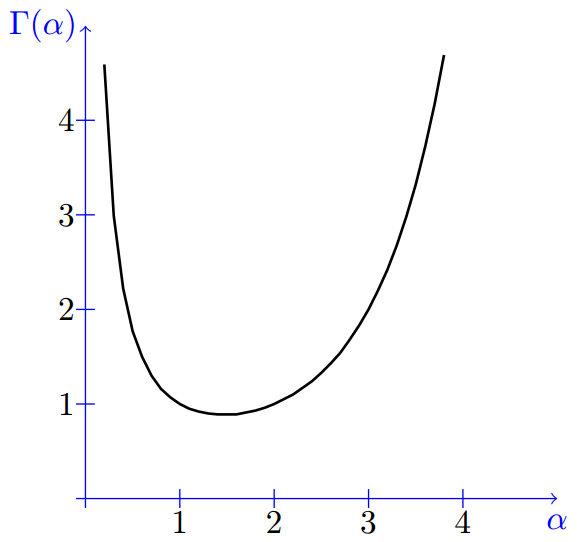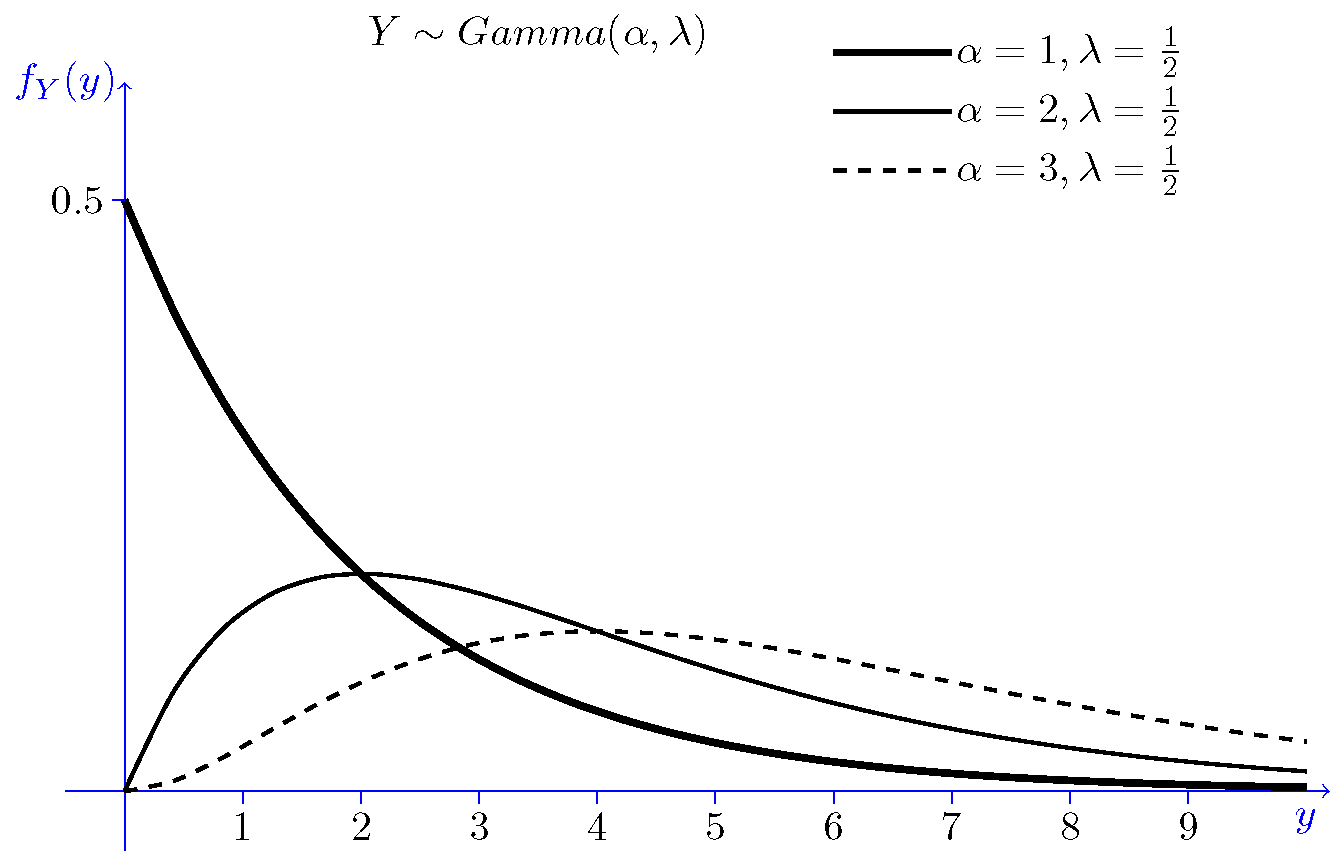## 4.2.4 Gamma Distribution

The gamma distribution is another widely used distribution. Its importance is largely due to its relation to exponential and normal distributions. Here, we will provide an introduction to the gamma distribution. In Chapters 6 and 11, we will discuss more properties of the gamma random variables. Before introducing the gamma random variable, we need to introduce the gamma function.

Gamma function: The gamma function , shown by $\Gamma(x)$, is an extension of the factorial function to real (and complex) numbers. Specifically, if $n \in \{1,2,3,...\}$, then $$\Gamma(n) = (n-1)!$$ More generally, for any positive real number $\alpha$, $\Gamma(\alpha)$ is defined as $$\Gamma(\alpha) = \int_0^\infty x^{\alpha - 1} e^{-x} {\rm d}x, \hspace{20pt} \textrm{for }\alpha>0.$$
Figure 4.9 shows the gamma function for positive real values.Figure 4.9: The Gamma function for some real values of $\alpha$.

Note that for $\alpha=1$, we can write \begin{align*} \Gamma(1) &= \int_0^\infty e^{-x} dx \\ &= 1. \end{align*} Using the change of variable $x = \lambda y$, we can show the following equation that is often useful when working with the gamma distribution: $$\Gamma(\alpha) = \lambda^{\alpha} \int_0^\infty y^{\alpha-1} e^{-\lambda y} dy \hspace{20pt} \textrm{for } \alpha,\lambda > 0.$$ Also, using integration by parts it can be shown that $$\Gamma(\alpha + 1) = \alpha\Gamma(\alpha), \hspace{20pt} \textrm{for } \alpha > 0.$$ Note that if $\alpha = n$, where $n$ is a positive integer, the above equation reduces to $$n! = n \cdot (n-1)!$$

### Properties of the gamma function

For any positive real number $\alpha$:
1. $\Gamma(\alpha) = \int_0^\infty x^{\alpha - 1} e^{-x} dx$;
2. $\int_0^\infty x^{\alpha - 1} e^{-\lambda x} dx = \frac{\Gamma(\alpha)}{\lambda^{\alpha}}, \hspace{20pt} \textrm{for } \lambda > 0;$
3. $\Gamma(\alpha + 1) = \alpha \Gamma(\alpha);$
4. $\Gamma(n) = (n - 1)!, \textrm{ for } n = 1,2,3,\cdots ;$
5. $\Gamma(\frac{1}{2}) = \sqrt{\pi}$.

Example
1. Find $\Gamma(\frac{7}{2}).$
2. Find the value of the following integral: $$I = \int_0^\infty x^{6} e^{-5x} dx.$$
• Solution
1. To find $\Gamma(\frac{7}{2}),$ we can write \begin{align} \Gamma(\frac{7}{2}) &= \frac{5}{2} \cdot \Gamma(\frac{5}{2}) \hspace{20pt} \textrm{(using Property 3)} \\ &= \frac{5}{2} \cdot \frac{3}{2} \cdot \Gamma(\frac{3}{2}) \hspace{20pt} \textrm{(using Property 3)} \\ &= \frac{5}{2} \cdot \frac{3}{2} \cdot \frac{1}{2} \cdot \Gamma(\frac{1}{2}) \textrm{(using Property 3)} \\ &= \frac{5}{2} \cdot \frac{3}{2} \cdot \frac{1}{2} \cdot \sqrt{\pi} \hspace{20pt} \textrm{(using Property 5)} \\ &= \frac{15}{8} \sqrt{\pi}. \end{align}
2. Using Property 2 with $\alpha = 7$ and $\lambda = 5$, we obtain \begin{align*} I &= \int_0^\infty x^{6} e^{-5x} dx \\ &= \frac{\Gamma(7)}{5^7} \\ &= \frac{6!}{5^7} \hspace{20pt} \textrm{(using Property 4)} \\ &\approx 0.0092 \end{align*}

Gamma Distribution:
We now define the gamma distribution by providing its PDF:
A continuous random variable $X$ is said to have a gamma distribution with parameters $\alpha > 0 \textrm{ and } \lambda > 0$, shown as $X \sim Gamma(\alpha,\lambda)$, if its PDF is given by $$f_X(x) = \left\{ \begin{array}{l l} \frac{\lambda^{\alpha} x^{\alpha-1} e^{-\lambda x}}{\Gamma(\alpha)} \hspace {5pt} x > 0\\ 0 \hspace{56pt} \textrm{otherwise} \end{array}\right.$$

If we let $\alpha = 1$, we obtain $$f_X(x) = \left\{ \begin{array}{l l} \lambda e^{-\lambda x} \hspace{20pt} x > 0\\ 0 \hspace{41pt} \textrm{otherwise} \end{array}\right.$$ Thus, we conclude $Gamma(1,\lambda) = Exponential(\lambda)$. More generally, if you sum $n$ independent $Exponential(\lambda)$ random variables, then you will get a $Gamma(n,\lambda)$ random variable. We will prove this later on using the moment generating function. The gamma distribution is also related to the normal distribution as will be discussed later. Figure 4.10 shows the PDF of the gamma distribution for several values of $\alpha$.Figure 4.10: PDF of the gamma distribution for some values of $\alpha$ and $\lambda$.

Example
Using the properties of the gamma function, show that the gamma PDF integrates to 1, i.e., show that for $\alpha , \lambda > 0$, we have $$\int_0^\infty \frac{\lambda^{\alpha}x^{\alpha - 1} e^{-\lambda x}}{\Gamma(\alpha)} dx = 1.$$
• Solution
• We can write \begin{align*} \int_0^\infty \frac{\lambda^{\alpha} x^{\alpha - 1} e^{-\lambda x}}{\Gamma(\alpha)} dx &= \frac{\lambda^{\alpha}}{\Gamma(\alpha)} \int_0^\infty x^{\alpha - 1} e^{-\lambda x} dx\\ \\ \hspace{20pt} &= \frac{\lambda^{\alpha}}{\Gamma(\alpha)} \cdot \frac{\Gamma(\alpha)}{\lambda^{\alpha}} \hspace{20pt} \textrm{(using Property 2 of the gamma function)}\\ \\ \hspace{0px} &= 1. \end{align*}

In the Solved Problems section, we calculate the mean and variance for the gamma distribution. In particular, we find out that if $X \sim Gamma(\alpha,\lambda)$, then $$EX = \frac{\alpha}{\lambda}, \hspace{20pt} Var(X) = \frac{\alpha}{\lambda^2}.$$

 The print version of the book is available on Amazon.Practical uncertainty: Userful Ideas in Decision-Making, Risk, Randomness, & AI